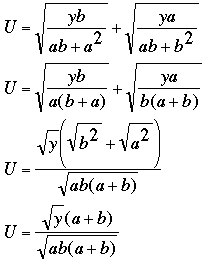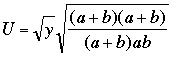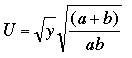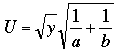SEARCH HOMEMath Central Quandaries & QueriesQuestion from Rubén, a teacher: I have the ecuation: U = [yb / (ab+a^2)]^1/2 + [ya / (ab+b^2)]^1/2 I know for sure this reduces to U = [y (1/a + 1/b)]^1/2 but I cannot find a way to get into that result! ThanksRubén wrote back that he solved this problem and sent us his solution. It is a nice exercise in manipulating algebraic expressions so I have posted his solution for others to see. Her is what Rubén sent us

Harley

I have solved the problem!!!Squared and root:thenConsidering (a+b)/ab = (1/a + 1/b)...RubénMath Central is supported by the University of Regina and The Pacific Institute for the Mathematical Sciences.